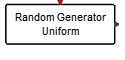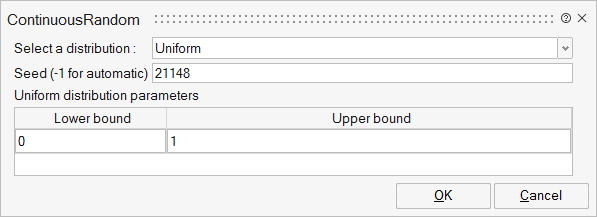# ContinuousRandom

This block is a continuous random wave generator. Each output component takes piecewise constant random values. Each time an event is received on the block input activation port, the outputs take new independent random values. The output port size is given by the size of the parameter matrices. You can select the distribution.SignalGenerators

## Description

The ContinuousRandom block is a continuous random wave generator. Each output component takes piecewise constant random values. Every time an event is received on the input event port, the outputs take new independent random values. Output port size is given by the size of the parameters matrices. You can choose the distribution.

Supported distributions are: Uniform, Normal, Lognormal, Beta, Gamma, Chi-squared, Exponential, F, T, Pearson I, Pearson III, Pearson V, Pearson VII, Inverse Gamma, Inverse Beta.

## ParametersNameLabelDescriptionData TypeValid Values

distrib

Select a distribution :

Structure

distrib/uniform

Uniform

Number

0
1

distrib/normal

Standard Normal

Number

0
1

distrib/log_normal

Log-Normal

Number

0
1

distrib/beta

Beta

Number

0
1

distrib/gamma

Gamma

Number

0
1

distrib/chi

Chi-squared

Number

0
1

distrib/exp

Exponential

Number

0
1

distrib/F

F-distribution

Number

0
1

distrib/T

T-distribution

Number

0
1

distrib/pearson

Pearson

Number

0
1

distrib/inv_gamma

Inverse Gamma

Number

0
1

distrib/inv_beta

Inverse Beta

Number

0
1

seed

Seed (-1 for automatic)

Seed for the random generator (scalar). seed must be an integer between 0 and 2^32-1. Negative value for seed means automatic selection.

Matrix

Uniform_param

Uniform distribution parameters

Structure

Uniform_param/A

Lower bound

Cell of matrices

Uniform_param/B

Upper bound

Cell of matrices

Normal_param

Normal distribution parameters

Structure

Normal_param/mean

Mean

Cell of matrices

Normal_param/variance

Standard deviation (&gt;0)

Cell of matrices

LNormal_param

Log-Normal distribution parameters

Structure

LNormal_param/meanL

Mean

Cell of matrices

LNormal_param/varianceL

Standard deviation (&gt;0)

Cell of matrices

Beta_param

Beta distribution parameters

Structure

Beta_param/alpha

First shape (Alpha) (&gt;0)

Cell of matrices

Beta_param/beta

Second shape (Beta) (&gt;0)

Cell of matrices

Gamma_param

Gamma distribution parameters

Structure

Gamma_param/k

Shape (K) (&gt;0)

Cell of matrices

Gamma_param/theta

Scale (Theta) (&gt;0)

Cell of matrices

Chi_param

Chi-squared distribution parameters

Structure

Chi_param/K

Degree of freedom

Cell of matrices

Exp_param

Exponential distribution parameters

Structure

Exp_param/lambda

Lambda (rate) (&gt;0)

Cell of matrices

F_param

F-distribution parameters

Structure

F_param/d1

Degrees of freedom 1 (&gt;0)

Cell of matrices

F_param/d2

Degrees of freedom 2 (&gt;0)

Cell of matrices

T_param

T-distribution parameters

Structure

T_param/t

Degree of freedom (&gt;0)

Cell of matrices

Pearson_param

Pearson distribution parameters

Structure

Pearson_param/mean

Mean

Cell of matrices

Pearson_param/variance

Variance (&gt;0)

Cell of matrices

Pearson_param/skewness

Skewness

Cell of matrices

Pearson_param/kurtosis

Kurtosis

Cell of matrices

Inv_gamma_param

Inverse-Gamma distribution parameters

Structure

Inv_gamma_param/alpha

Shape (Alpha) (&gt;0)

Cell of matrices

Inv_gamma_param/beta

Scale (Beta) (&gt;0)

Cell of matrices

Inv_beta_param

Inverse-Beta distribution parameters

Structure

Inv_beta_param/alpha

First shape (Alpha) (&gt;0)

Cell of matrices

Inv_beta_param/beta

Second shape (Beta) (&gt;0)

Cell of matrices

## Ports

NameTypeDescriptionIO TypeNumber

Port 1

explicit

output

1

Port 2

activation

input

1

NameValueDescription

always active

no

Time Dependency = standard mode activated

direct-feedthrough

no

zero-crossing

no

mode

no

continuous-time state

no

discrete-time state

yes